Proceedings of STeP'96. Jarmo Alander, Timo Honkela and Matti Jakobsson (eds.),
Publications of the Finnish Artificial Intelligence Society, pp. 13-24.

# Turing Machines are Recurrent Neural Networks

Heikki Hyötyniemi
Helsinki University of Technology,
Control Engineering Laboratory
Otakaari 5A, FIN-02150 Espoo, Finland

### Abstract:

Any algebraically computable function can be expressed as a recurrent neural network structure consisting of identical computing elements (or, equivalently, as a nonlinear discrete-time system of the form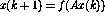, where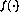is a simple `cut' function). A constructive proof is presented in this paper.

A PostScript version of this paper is available also at
http://www.hut.fi/~hhyotyni/HH1/HH1.ps

# 1 Introduction

## 1.1 Neural networks in classification

Neural networks can be used, for example, for classification tasks, telling whether an input pattern belongs to a specific class or not. It has been known for a long time that one-layer feedforward networks can be used to classify only patterns that are linearly separable---the more there are successive layers, the more complex the distribution of the classes can be.

When feedback is introduced in the network structure, so that perceptron output values are recycled, the number of successive layers becomes, in principle, infinite. Is the computational power enhanced qualitatively? The answer is yes. For example, it is possible to construct a classifier for telling whether the input integer is a prime number or not. It turns out that the size of the network for this purpose can be finite, even if the input integer size is not limited, the number of primes that can be correctly classified being infinite.

In this paper, it is shown that a recurrent network structure consisting of identical computing elements can be used to accomplish any (algorithmically) computable function.

According to the basic axiom of computability theory, computable functions can be implemented using a Turing machine. There are various ways to realize a Turing machine.

Define the program language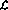. There are four basic operations in this language:

```No operation: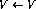Increment: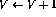Decrement: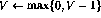Conditional branch: 		  IF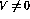GOTO j.

```
Here, V stands for any variable having positive integer values, and j stands for any line number. It can be shown that if a function is Turing computable, it can be coded using this simple language (for details, see ).

# 2 `Turing network'

## 2.1 Recurrent neural network structure

The neural network to be studied now consists of perceptrons, all having identical structure. The operation of the perceptron number q can be defined as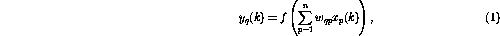where the perceptron output at the current time instant, denoted by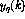, is calculated using the n inputs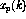,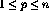. The nonlinear function f is now defined (somewhat exceptionally) as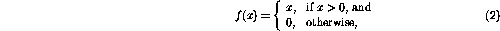so that the function simply `cuts off' the negative values. The recurrence in the perceptron network means that perceptrons can be combined in complex ways. In Fig. 1, the recurrent structure is shown in a common framework: now,, and n is the number of perceptrons. The connection from perceptron p to perceptron q is represented by the scalar weigth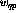in (1).

Given some initial state, the network state is iterated until no more changes take place. The result can be read in that steady state, or `fixed point' of the network.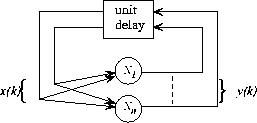Figure 1:   The overall outlook of the recurrent network structure. The structure is autonomous with no external input---the behavior of the network is determined exclusively by the initial state

## 2.2 Construction of the network

It is now shown how the programcan be implemented in a perceptron network. The network consists of the following nodes (or perceptrons):

• For each variable V in the program, there is a variable node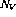.
• For each program row i, there is an instruction node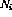.
• For each conditional branch instruction on row i, there are, additionally, two transition nodes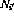and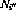.
A realization of a program in languageconsists of the following changes in the perceptron network:
• For each variable V in the program, augment the network with the following link: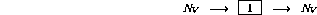• If there is no operation () on row i of the program code then augment the network with the following link (assuming that node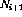exists):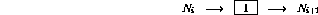• If there is an increment operation () on row i then augment the network as follows: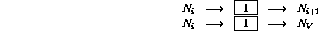• If there is a decrement operation () on row i then augment the network as follows: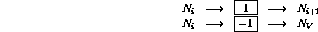• If there is a conditional branch ( IFGOTO j) on row i then augment the network as follows: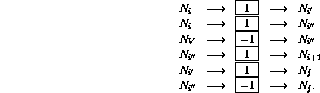## 2.3 Proof of equivalence

It needs to be shown that the internal state of the network, or the contents of the network nodes, can be identified with the program states, and the succession of the network states corresponds to the program flow.

Define a `legal state' of the network as follows:

• all transition nodesand(as defined in 2.2) have zero outputs (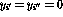);
• at most one of the instruction nodeshas unit output (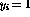), all other having zero outputs, and
• variable nodes have nonnegative integer output values.
If all of the instruction nodes have zero outputs, the state is final. A legal state of the network can directly be interpreted as a program `snapshot'---if, the program counter is on the line i, and the corresponding variable values are stored in the variable nodes.

The changes in the network state are activated by the non-zero nodes. First, concentrating on the variable nodes, it turns out that they behave as integrators, the previous contents of the node being circulated back to the same node. The only connections from variable nodes to other nodes have negative weights---that is why, nodes containing zeros will not be changed, because of the nonlinearity (2).

Next, instruction nodes are elaborated on. Assume that the only non-zero instruction node isat time k---this corresponds to the program counter being on row i in the program code.

If the row i in the program is, the one step ahead behavior of the network can be expressed as (only showing the nodes that are affected)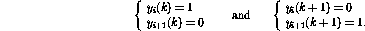It turns out that the new network state is again legal. Comparing to the program code, this corresponds to the program counter being transferred to row i+1.

On the other hand, if the row i in the program is, the one step ahead behavior is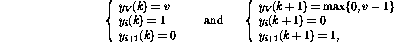so that, in addition to transferring the program counter to the next line, the value of the variable V is decremented. The operation of the network, if the row i is, will be the same, with the exception that the value of the variable V is incremented.

The conditional branch operation ( IFGOTO j) on row i activates a more complex sequence of actions: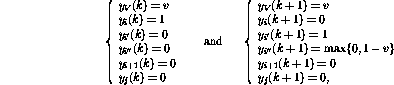and, finally,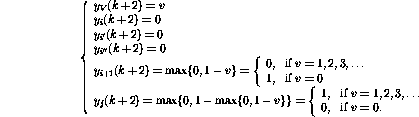It turns out that after these steps the network state can again be interpreted as another program snapshot. The variable values have been changed and the token has been transferred to a new location just as if the corresponding program line were executed. If the token disappears, the network state does no more change---this can happen only if the program counter goes `outside' the program code, meaning that the program terminates. The operation of the network is also similar to the operation of the corresponding program, and the proof is completed.

# 3 Modifications

## 3.1 Extensions

It is easy to define additional streamlined instructions that can make the programming easier, and the resulting programs more readable and faster to execute. For example,

• An unconditional branch ( GOTO j) on row i can be realized as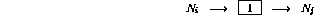• Addition of a constant c to a variable (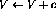) on row i can be realized as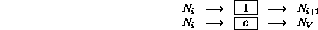• A different kind of conditional branch ( IF V=0 GOTO j) on row i can be realized as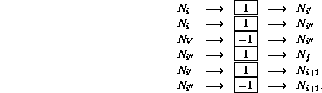• Additionally, various increment/decrement instructions can be evaluated simultaneously. Assume that the following operations are to be executed: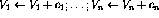. Only one nodeis needed: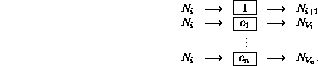The above approach is by no means the only way to realize the Turing machine. It is a straightforward implementation that is not necessarily optimal in applications.

## 3.2 Matrix formulation

The above construction can also be implemented in a matrix form. The basic idea is to store the variable values and the `program counter' in the process state s, and let the state transition matrix A stand for the links between the nodes. The operation of the matrix structure can be defined as a discrete-time dynamic process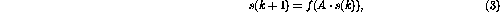where the nonlinear vector-valued functionis now defined elementwise as in (2). The contents of the state transition matrix A are easily decoded from the network formulation---the matrix elements are the weights between the nodes.

This matrix formulation resembles the `concept matrix' framework that was presented in .

# 4 Example

Assume that a simple function y=x is to be realized, that means, the value of the input variable x should be transferred to the output variable y. Using languagethis can be coded as (letting the `entry point' now be not the first but the third row):

```
back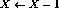row 1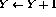row 2

start 		  IF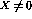GOTO back 		  row 3

```
The resulting perceptron network is presented in Fig. 2. Solid links represent positive connection (weights being 1), and dashed links represent negative connection (weights -1). Compared to Fig. 1, the network structure is redrawn, and it is simplified by integrating the delay elements in the nodes.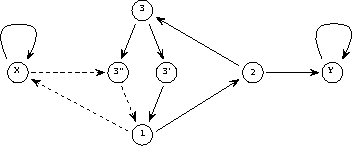Figure 2:   Network realization of the simple program

In the matrix form the above program looks like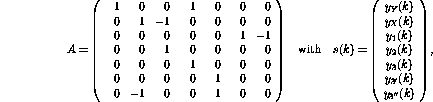the first two rows/columns in the matrix A corresponding to the links connected to the nodes that stand for the two variables Y and X, the next three standing for the three program lines (1, 2, and 3), and the last two standing for the additional nodes (3' and 3'') needed for the branch instruction.

The initial (before iteration) and final (after iteration, when the fixed point is found) states are then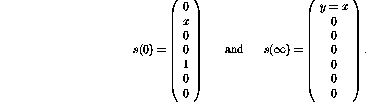If the values of the variable nodes would remain strictly between 0 and 1, the operation of the dynamic system (3) would be linear, the functionhaving no effect at all. In principle, linear systems theory could then be utilized in the analysis. For example, in Fig. 3, the eigenvalues of the state transition matrix A are shown. Even if there are eigenvalues outside the unit circle in the above example, the nonlinearity makes the iteration always stable. It turns out that the iteration always converges after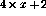steps, where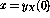.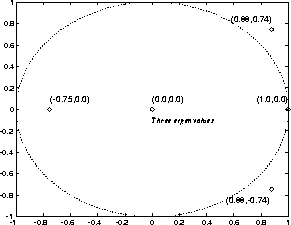Figure 3:   `Eigenvalues' of the simple program

# 5 Discussion

## 5.1 Theoretical aspects

It was shown that a Turing machine can be coded as a perceptron network. By definition, all computable functions are Turing computable---in the framework of computability theory, there cannot exist a more powerful computing system. That is why, it can be concluded that

The recurrent perceptron network (as presented above) is (yet another) form of a Turing machine.
The nice thing about this equivalence is that results of computability theory are readily available---for example, given a network and an initial state, it is not possible to tell whether the process will eventually stop or not.

The above theoretical equivalence does not tell anything about the efficiency of the computations. As compared to traditional Turing machine implementations (today's computers, actually), the different mechanisms that take place in the network can make some functions better realizable in this framework. At least in some cases, for example, the network realization of an algorithm can be parallelized by allowing multiple `program counters' in the snapshot vector. The operation of the network is strictly local rather than global. An interesting question arises, for example, whether the class of NP-complete problems could be attacked more efficiently in the network environment!

Compared to the language, the network realization has the following `extensions':

• The variables can be continuous, not only integer valued. Actually, the (theoretical) capability of presenting real numbers makes the network realization more powerful than the languageis, all numbers presented in the language being only rational.
• There can exist various `program counters' simultaneously, and the transfer of control may be `fuzzy', meaning that program counter values presented by the instruction nodes may be non-integers.
• A minor extension is the freely definable program entry point. This may facilitate simpler programs---for example, the copying of a variable was done above in three program lines, while the nominal solution (see [1, p. 17,]) takes seven lines and an additional local variable.
Compared to the original program code, the matrix formulation is a clearly more `continuous' information representation formalism than the program code is---the parameters can (often) be modified without the iteration result abruptly changing. This `redundancy' can perhaps be utilized in some applications. For example, when using Genetic Algorithms (GA) for structural optimization, the stochastic search strategy that is used in the genetic algorithms can be made more efficient: after a change in the system structure, the local minimum of the continuous cost function can be searched using some traditional technique (see ).

Learning a finite state machine structure by examples, as presented in , suggests an approach to iteratively enhancing the network structure also in this more complex case.

It is not only the neural networks theory that may benefit of the above result---looking merely at the dynamic system formulation (3), it becomes evident that all of the phenomena found in the field of computability theory are also present in simple-looking nonlinear dynamic processes. The undecidability of the halting problem, for example, is an interesting contribution in the field of systems theory: for any decision procedure, represented as a turing machine, there exist dynamic systems of the form (3) that defies this procedure---for example, no general purpose stability analysis algorithms can be constructed.

## 5.2 Related work

There are some similarities between the presented network structure and the recurrent Hopfield neural network paradigm (for example, see ). In both cases, the `input' is coded as the initial state in the network, and the `output' is read from the final state of the network after iteration. The fixed points of the Hopfield network are the preprogrammed pattern models, and the inputs are `noisy' patterns---the network can be used to enhance the corrupted patterns. The outlook of the nonlinear functionin (2) makes the number of possible states in the above `Turing network' infinite. As compared with Hopfield networks, where the unit outputs are always -1 or 1, it can be seen that, theoretically, these network structures are very different. For example, while the set of stable points in a Hopfield network is finite, a program, represented by the Turing network, typically has an unbounded number of possible outcomes. The computational power of Hopfield networks is discussed in .

Petri nets are powerful tools in modeling of event-based and concurrent systems . Petri nets consist of places and transitions, and arcs connecting them. Each of the places may contain an arbitrary number of tokens, the distribution of tokens being called a marking of a Petri net. If all input places of a transition are occupied by tokens, the transition may fire, one token being deleted from each of the input places and one token being added to each of its output places. It can be shown that an extended Petri net with additional inhibitory arcs also has the power of a Turing machine (see ). The main difference between the above Turing networks and the Petri nets is that the Petri net framework is more complex, with specially tailored constructs, and it cannot be expressed in the simple general form (3).

# Acknowledgement

This research has been financed by the Academy of Finland, and this support is gratefully acknowledged.

## References

1
Davis, M. and Weyuker, E.: Computability, Complexity, and Languages---Fundamentals of Theoretical Computer Science. Academic Press, New York, 1983.

2
Haykin, S.: Neural Networks. A Comprehensive Foundation. Macmillan College Publishing, New York, 1994.

3
Hyötyniemi, H.: Correlations---Building Blocks of Intelligence? In Älyn ulottuvuudet ja oppihistoria (History and dimensions of intelligence), Finnish Artificial Intelligence Society, 1995, pp. 199--226.

4
Hyötyniemi, H. and Koivo, H.: Genes, Codes, and Dynamic Systems. In Proceedings of the Second Nordic Workshop on Genetic Algorithms (NWGA'96), Vaasa, Finland, August 19--23, 1996.

5
Manolios, P. and Fanelli, R.: First-Order Recurrent Neural Networks and Deterministic Finite State Automata. Neural Computation 6, 1994, pp. 1155--1173.

6
Orponen, P.: The Computational Power of Discrete Hopfield Nets with Hidden Units. Neural Computation 8, 1996, pp. 403--415.

7
Peterson, J.L.: Petri Net Theory and the Modeling of Systems. Prentice--Hall, Englewood Cliffs, New Jersey, 1981.

Turing Machines are Recurrent Neural Networks

This document was generated using the LaTeX2HTML translator Version 95.1 (Fri Jan 20 1995) Copyright © 1993, 1994, Nikos Drakos, Computer Based Learning Unit, University of Leeds.

The command line arguments were:
latex2html -split 0 -show_section_numbers HH1.tex.

The translation was initiated by Heikki Hy|tyniemi on Mon Aug 26 12:40:58 EET DST 1996

...Networks
This paper was presented at the Symposium on Artificial Networks (Finnish Artificial Intelligence Conference), August 19--23, in Vaasa, Finland, organized by the Finnish Artificial Intelligence Society and University of Vaasa. Published in "STeP'96---Genes, Nets and Symbols", edited by Alander, J., Honkela, T., and Jakobsson, M., Finnish Artificial Intelligence Society, pp. 13--24.
```
```
...nodes
A simple `compiler' between the language 60#60 and the matrix A, written in Matlab, is available from the author
```
```

Heikki Hy|tyniemi
Mon Aug 26 12:40:58 EET DST 1996